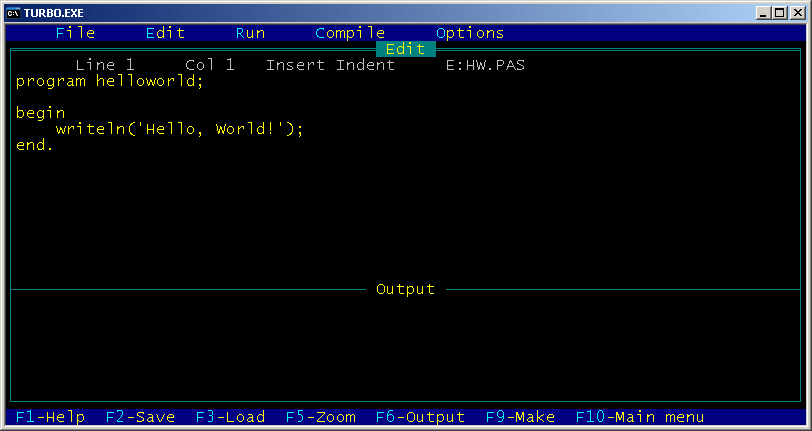# Turbo Pascal 4.0

Version of implementation Turbo Pascal of programming language Pascal

Turbo Pascal 4.0 was the fourth version of Turbo Pascal series, released on November 2, 1987.

Turbo Pascal 4.0 introduced drastic changes in the compiler: it stopped supporting CP/M operating systems (which were out of date by 1987) and compiled programs to .EXE files instead of simpler .COM ones. Other changes included support of EGA/VGA graphics, added `longint` data type and extended set of examples.

The language changed as well: this version introduced major concept of program units, i.e., libraries of procedures which could be compiled separately and used later.

Interface changed just as much as the compiler: it turned to full-screen editor with drop-down menu on the top and output at the bottom of the screen. The environment provided much more possibilities and options than in previous versions: one could set lots of compiler options, environment options (like automatic save and backup of edited files, tab size etc.), working directories and parameters of command line (for programs which required them).Turbo Pascal 4.0: general view

## Examples:

### Fibonacci numbers - Pascal (58):

This example uses recursive definition of Fibonacci numbers.

``````program fibonacci;

function fib(n:integer): integer;
begin
if (n <= 2) then
fib := 1
else
fib := fib(n-1) + fib(n-2);
end;

var
i:integer;

begin
for i := 1 to 16 do
write(fib(i), ', ');
writeln('...');
end.
``````

### Hello, World! - Pascal (57):

``````program helloworld;

begin
writeln('Hello, World!');
end.
``````

### Factorial - Pascal (96):

This example is exactly the same as main recursive example for Pascal implementations, except for that it uses `real` data type to store factorial values. Command `writeln(f:-1:0)` outputs the floating point number `f` with 0 digits after decimal point and left-justifies it.

``````program factorial;

function fact(n: integer): real;
begin
if (n = 0) then
fact := 1
else
fact := n * fact(n - 1);
end;

var
n: integer;

begin
for n := 0 to 16 do
writeln(n, '! = ', fact(n):-1:0);
end.
``````

### Factorial - Pascal (44):

This example uses recursive factorial definition.

Note that this example works in all given implementations of Pascal, but it produces slightly different results. In gpc everything works perfectly. Turbo Pascal and Free Pascal have arithmetic overflow for factorial of numbers greater than 12, but Free Pascal reports an error:

13! = Runtime error 215 at \$004013C7
\$004013C7
\$00401449
\$004063E0

while Turbo Pascal doesn’t detect the error and simply prints wrong values:

13! = 1932053504
14! = 1278945280
15! = 2004310016
16! = 2004189184

This example doesn’t work in Turbo Pascal 3.0 and earlier due to absence of `longint` data type in these versions.

In GNU Pascal this program works without any problems.

``````program factorial;

function fact(n: integer): longint;
begin
if (n = 0) then
fact := 1
else
fact := n * fact(n - 1);
end;

var
n: integer;

begin
for n := 0 to 16 do
writeln(n, '! = ', fact(n));
end.
``````

### Quadratic equation - Pascal (178):

Pascal has built-in complex data type `complex`, but using it is inconvenient in this case, because `writeln` can’t output complex numbers directly, and functions `Re` and `Im` would have to be used. In this example calculations are done in real numbers. Library function `halt` (added in Extended Pascal) exits current block (in later versions it is replaced with `exit`).

``````program Quadratic;

var
A,B,C,D: integer;

begin
write('A = ');
if (A=0) then
begin
halt;
end;
write('B = ');
write('C = ');
D := B*B-4*A*C;
if (D=0) then
begin
writeln('x = ',-B/2.0/A);
halt;
end;
if (D>0) then
begin
writeln('x1 = ',(-B+Sqrt(D))/2.0/A);
writeln('x2 = ',(-B-Sqrt(D))/2.0/A);
end
else
begin
writeln('x1 = (',-B/2.0/A,',',Sqrt(-D)/2.0/A,')');
writeln('x2 = (',-B/2.0/A,',',-Sqrt(-D)/2.0/A,')');
end;
end.
``````

### CamelCase - Pascal (284):

This example processes the string char by char, and works with ASCII-codes to figure out whether they are lower- or uppercase letters. `ord` returns ASCII-code of a character, while `chr` converts given ASCII-code into a character. String capacity is omitted and thus set to 255 by default.

Note that in Turbo Pascal series this program works only with Turbo Pascal 4.0 and higher due to the fact that earlier versions didn’t have `char` datatype.

``````program Camelcase;

var
text, cc: string;
c: char;
i: integer;
lastSpace: boolean;

begin
lastSpace := true;
cc := '';
for i := 1 to Length(text) do
begin
c := text[i];
if ((c >= #65) and (c <= #90)) or ((c >= #97) and (c <= #122)) then
begin
if (lastSpace) then
begin
if ((c >= #97) and (c <= #122)) then
c := chr(ord(c) - 32);
end
else
if ((c >= #65) and (c <= #90)) then
c := chr(ord(c) + 32);
cc := cc + c;
lastSpace := false;
end
else
lastSpace := true;
end;
writeln(cc);
end.
``````

### CamelCase - Pascal (285):

This example is similar to previous one, but uses sets of characters for letter check. This makes the code more readable.

Note that in Turbo Pascal series this program works only with Turbo Pascal 4.0 and higher due to the fact that earlier versions didn’t have `char` datatype.

``````program Camelcase;

var
text, cc: string;
c: char;
i: integer;
lastSpace: boolean;
upper, lower: set of char;

begin
upper := ['A'..'Z'];
lower := ['a'..'z'];
lastSpace := true;
cc := '';
for i := 1 to Length(text) do
begin
c := text[i];
if (c in lower) or (c in upper) then
begin
if (lastSpace) then { convert to uppercase }
begin
if (c in lower) then
c := chr(ord(c) - 32);
end
else { convert to lowercase }
if (c in upper) then
c := chr(ord(c) + 32);
cc := cc + c;
lastSpace := false;
end
else
lastSpace := true;
end;
writeln(cc);
end.
``````